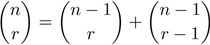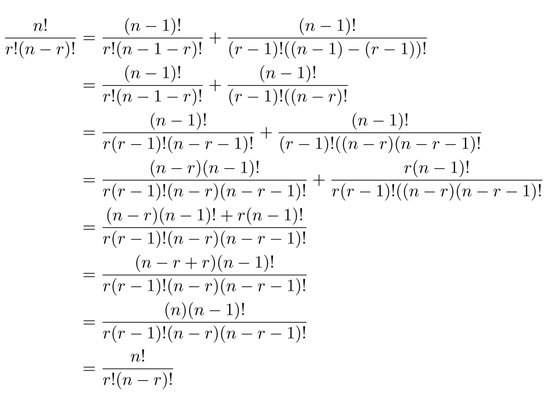SEARCH HOMEMath Central Quandaries & QueriesQuestion from fae, a student: Prove that ( n ) = ( n – 1) + ( n - 1 ) ( r )         ( r )        (r-1) NOTE: the ( ) should be one for n taken r and so on. but there is no one big ( ) that will cater two linesHi Fae,
I assume this what you are trying to prove:When working with proofs it is usually best to try to simplify the more complicated side. I will solely manipulate the right side of the proof.\

If we need to write both sides in terms of the definition of a combination to be able to manipulate the proof. If we consider the meaning of a factorial like (n-r)!=(n-r)(n-r-1)(n-r-2)...(2)(1) we can change (n-r)! to (n-r)(n-r-1)!. This trick will allows us to find a much simpler common denominator. Once the fractions are added and simplified, this same trick allows use to complete our proof.Let us know if you any further questions about the proof.
JaniceMath Central is supported by the University of Regina and The Pacific Institute for the Mathematical Sciences.